# Rotation - think pencil compass

On any mathematical rotation think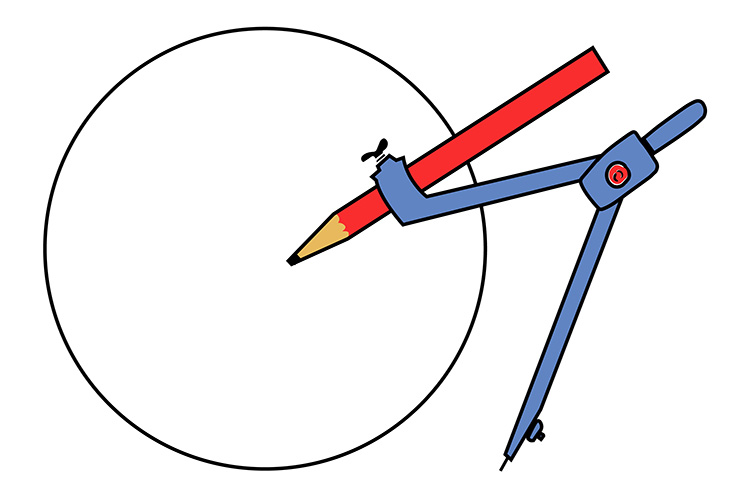Rotation - Pencil compass

And with any rotation a pencil compass would need a centre, the direction to move (clockwise or anticlockwise) and the number of degrees to move.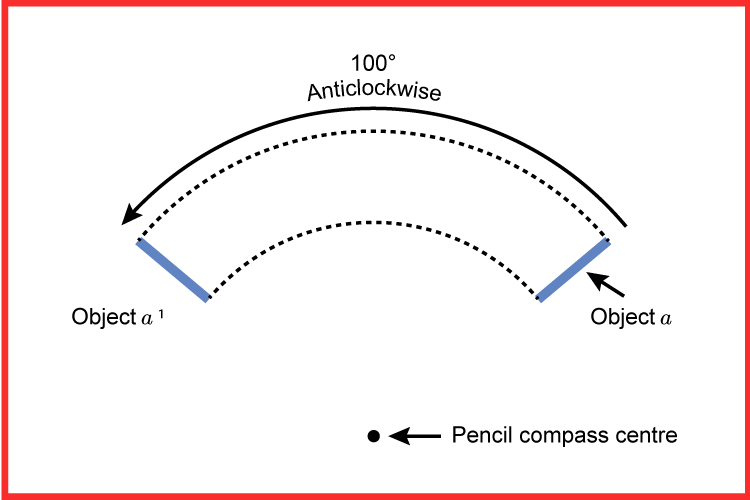Example

Below is an example of a triangle (W). Rotate this triangle 90° clockwise with the centre of rotation being at the origin of the graph (0,0).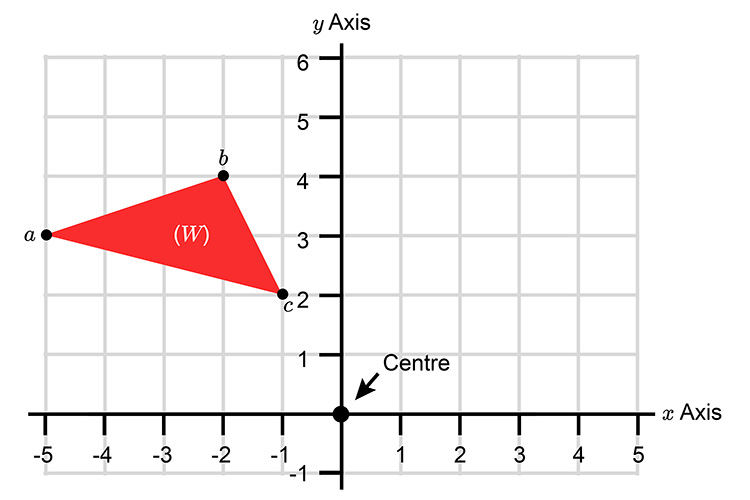Rotate point "a" first.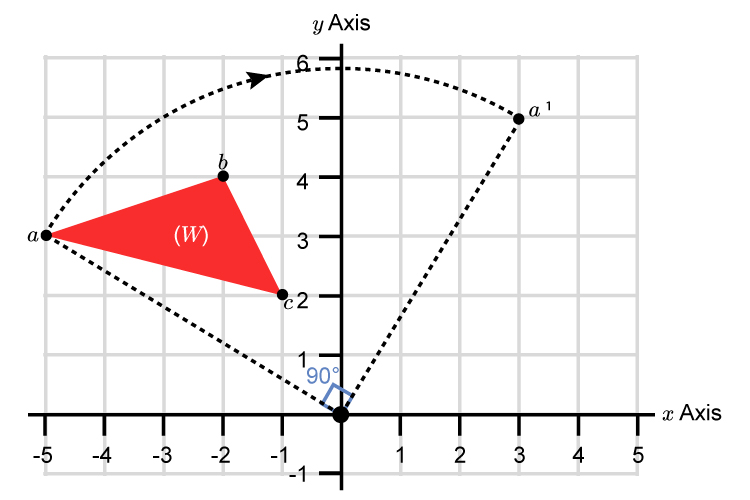Now rotate point "b"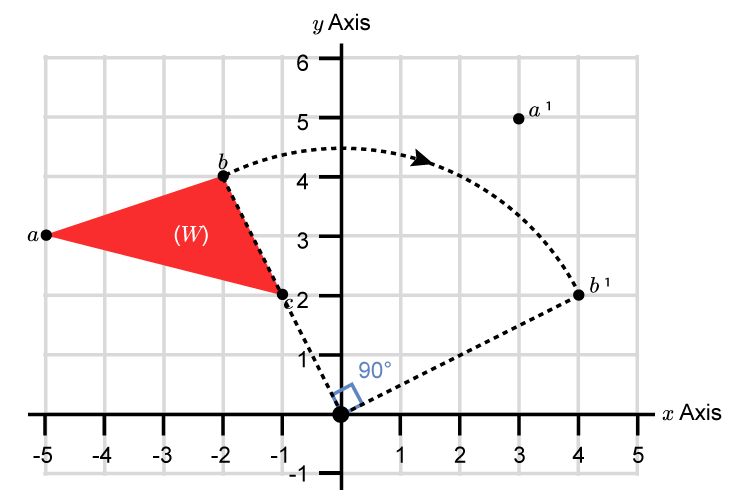Finally rotate point "c"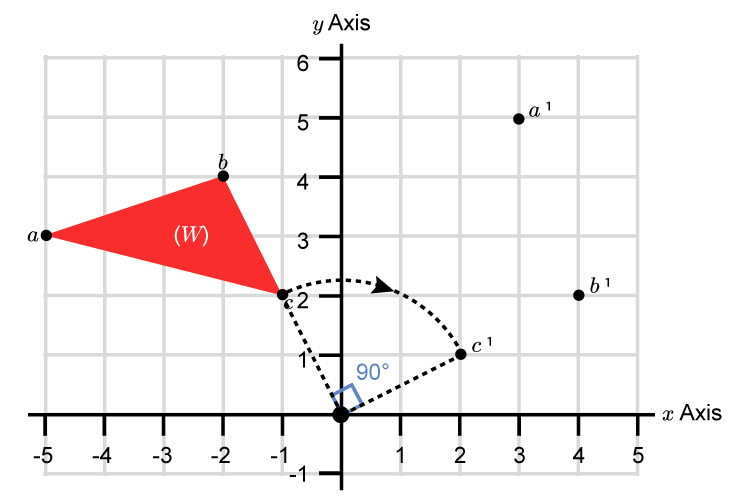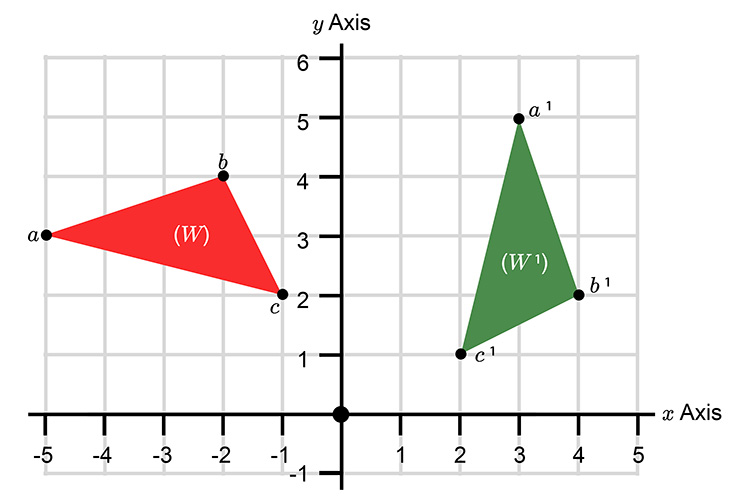Triangle W rotated 90° clockwise from (0,0)=W^1.